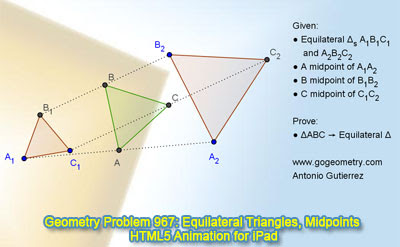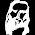## Monday, January 27, 2014

### Dynamic Geometry Problem 967: Three Equilateral Triangles, Midpoints.

Geometry Problem. GeoGebra, HTML5 Animation for iPad and Nexus.
Post your solution in the comments box below.

Level: Mathematics Education, High School, Honors Geometry, College.

Click the figure below to see the dynamic geometry demonstration of problem 967.#### 5 comments:

1.Let z(P) be the complex number representing P.
Let ω=cos(2π/3)+i sin(2π/3).
Let [z(P), z(Q), z(R)]=z(P)+ω z(Q)+ω² z(R)

Then we have
[z(A₁), z(C₁), z(B₁)] = [z(A₂), z(C₂), z(B₂)] = 0

Hence,
[z(A), z(C), z(B)] = 1/2 ( [z(A₁), z(C₁), z(B₁)] + [z(A₂), z(C₂), z(B₂)] ) = 0

Thus ABC is equilateral triangle.

1.To Jacob
I have 2 comments about your solution:
1. if the origin of complex plan is located at center of triangle A1B1C1 then we have Z(A1)+Z(B1)+Z(C1)= 0 but Z(A2)+Z(B2)+Z(C2) not equal 0 in general case. please explain.

2. Assume that we have Z(A)+Z(B)+Z(C)= 0 . This mean that origin of complex plan is located at centroid of triangle ABC . It doesn't mean that triangle ABC is equilateral.
Peter Tran

2.To Peter,

The expression I am using is z(A₁)+ω z(C₁)+ω² z(B₁)=0, but not z(A₁)+z(C₁)+z(B₁)=0.
So there is no conflict.

Jacob

2.Good proof!
What if two triangles have opposite orientations?

3.Let O is the center of spiral similarity to transform equilateral triangle A1B1C1 to A2B2C2
We will have ∆A1OA2 ~ ∆C1OC2~ ∆B1OB2… (properties of spiral similarity transformation)
With medians OA, OB, OC
Due to similarity of these triangles we also have
1. ∠ (A1OA)= ∠ (C1OC)= ∠ (B1OB)= θ … (angles forms between corresponding sides to corresponding medians)
2. OA/OA1=OB/OB1=OC/OC1= k …( ratio of corresponding median to corresponding sides)
So triangle ABC will be the image of triangle A1B1C1 in the spiral similarity transformation ( center O, angle of rotation= θ and factor of dilation = k)
Since A1B1C1 is equilateral so the image ABC be equilateral